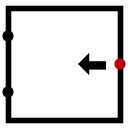#Bitschieber

 Bibliothek: Arithmetic Eingeführt: 2.3.0 Aussehen:## Verhalten

This component includes two inputs, data and dist, and it has one output, which is the result of shifting data by dist places. Both data and output have the same number of bits in them. The component supports the following shift types:

• Logical Left: All bits in data are shifted up dist places, with the bottom dist places filled with 0's. For example, 11001011 logically shifted left twice is 00101100. (The top two ones are lost.)
• Logical Right: All bits in data are shifted down dist places, with the upper dist places filled with 0's. For example, 11001011 logically shifted right twice is 00110010. (The bottom two ones are lost.)
• Arithmetic Right: All bits in data are shifted down dist places, with the upper dist places filled with repetitions of whatever the uppermost bit in data. For example, 11001011 arithmetically shifted right twice is 11110010.
• Roll Left: All bits in data are shifted up dist places, with the top dist places wrapped around into the bottom. For example, 11001011 rolled left twice is 00101111.
• Roll Right: All bits in data are shifted down dist places, with the bottom dist places wrapped around into the top. For example, 11001011 rolled right twice is 11110010.

Note that if dist contains any floating or error inputs, then the output is composed entirely of error values, since there is no way to guess how far to shift the input.

## Pins

West edge, north end (input, bit width matches the Data Bits attribute)
The value to be shifted.
West edge, south end (input, bit width is computed as below)
The number of bits by which to shift the data input. This input should have as many bits as is the minimum number to indicate any shift distance from 0 up to one less than Data Bits; that is, it should be the ceiling of the base-2 logarithm of Data Bits. For example, if Data Bits were 8, this input would require 3 bits; but if it were 9, it would require 4 bits.
East edge (output, bit width matches the Data Bits attribute)
The result of shifting the input value by the input distance.

## Attribute

When the component is selected or being added, Alt-0 through Alt-9 alter its Data Bits attribute.

Data Bits
The bit width of the data input and of the output.
Shift Type
One of the five possible shift types as outlined above (Logical Left, Logical Right, Arithmetic Right, Roll Left, Roll Right).

Keines.

## Verhalten des Textwerkzeugs

Keines.

Zurück zur Bibliotheksreferenz